# Plus Two Computer Science Chapter Wise Questions and Answers Chapter 1 Structures and Pointers

Students can Download Chapter 1 Structures and Pointers Questions and Answers, Plus Two Computer Science Chapter Wise Questions and Answers helps you to revise the complete Kerala State Syllabus and score more marks in your examinations

## Kerala Plus Two Computer Science Chapter Wise Questions and Answers Chapter 1 Structures and Pointers

### Plus Two Computer Science Structures and Pointers One Mark Questions and Answers

Question 1.
Name a method used to put logically related elements in one unit.
Structure or class

Question 2.
…………… keyword is used to specify a structure.
struct.

Question 3.
State True or False.
‘A structure can be assigned to another structure only if both the structures are of same structure type’.

Question 4.
A structure brings together a group of
(a) Items of the same data type
(b) Integers with user defined names.
(c) Related data items and functions, if needed.
(d) None of the above
(c) Related data items and functions, if needed.

Question 5.
Pick the odd one out from the following.
(a) int
(b) struct
(c) char
(d) long
(b) structQuestion 6.
Select the structure member operator. Structure member operator select
(a) *
(b) .
(c) ®
(d) [ ]
(b) .

Question 7.
The address of a computer memory starts at ………
(a) 0
(b) 1
(c) 1024
(d) none of these
(a) 0

Question 8.
The area used for dynamic memory allocation is called
(a) heap
(b) stack
(c) RAM
(d) Hard Disk
(a) heap

Question 9.
Given int *P, x = 35; P = &x; what is the value of *P?
(a) 34
(b) 35
(c) 0
(d) none of these
(b) 35

Question 10.
int *P; p is having the address 2340. What will be the value of P after the execution of the statements P++; P = P – 3;
(a) 2340
(b) 2342
(c) 2338
(d) 2332
(d) 2332Question 11.
Dynamic allocation means memory allocation at the time of ……….
(a) compilation
(b) debugging
(c) execution
(d) programming
(c) execution

Question 12.
The ‘new’ operator should be matched with a ……….
(a) pointer
(b) reference
(c) delete
(c) delete

Question 13.
In the declaration void ff (int &, float &); the parameters are passed by …….
(a) reference
(b) value
(c) pointers
(d) default
(a) reference

Question 14.
Using ……… the memory location of a variable can be directly accessed and manipulated.
Pointers.

Question 15.
Anil argued that memory can be allocated as and when required during runtime. Do you agree with this opinion? Justify.
Yes. Memory can be allocated during run time using the operator new.Question 16.
Name the unary operator that returns the address of its operand.

Question 17.
Which among the following operators cannot be used with pointers?
(a) +
(b) –
(c) /
(d) None of these
(c) /

Question 18.
The address of the first byte is known as
(a) start
(d) none of these

Question 19.
What are the free store operators?
‘new’ and ‘delete’ operators.

Question 20.
Give the syntax of new operator.
= new ;
eg: int *P = new int;Question 21.
What does the following stmt mean?
int *P = new int ;
Allocating memory for an integer array of 15 elements.

Question 22.
The ……. operator reverses the action of the ‘new’ operator.
delete.

Question 23.
Which operator is used to access individual elements of a structure ora class while using pointer variables?
(a) *
(b) .
(c) ?
(d) None of these
(b) .

Question 24.
A pointer is
(a) the address of a variable
(b) an indication of the variable to be accessed next
(c) a variable for storing addresses
(d) the datatype of an address variable
(c) a variable for storing addresses

Question 25.
The expression * test can be said to ‘test
(a) be a ponterto test
(b) refer to the contents of test
(c) dereference test
(d) refer to the value of the variable pointed to by test
(d) refer to the value of the variable pointed to by testQuestion 26.
The expression double * can be said to double*
(a) be a pointer pointing to variable double
(b) refer to contents of double
(c) be a pointer to a double type value
(d) refer to the value of the variable pointed to by double.
(c) be a pointer to a double type value

Question 27.
The new operator –
(a) returns a pointer to a variable
(b) creates a variable called new
(c) obtains memory for a new variable
(d) tells how much memory is available
(c) obtains memory for a new variable

Question 28.
Write a declaration for an array of 8 pointers to float float values
(a) float a []
(b) float*[*a] 
(c) float *a
(d) float [*a]
(b) float*[*a] 

Question 29.
A pointer to void can hold pointers to ………….
(a) void
(b) int
(c) float
(d) any data type
(d) any data type

Question 30.
The contents of two pointers that point to adjacent variable of type float differ by
(a) 4
(b) 2
(c) 1
(d) 8
(a) 4

Question 31.
In the …….. memory allocation, the amount of memory to be allocated is not known beforehand
(a) Static
(b) binding
(c) object
(d) dynamic
(d) dynamicQuestion 32.
Improper use of ‘new’ and ‘delete’ may lead to ….. ‘new’, ‘delete’ operator
(a) increase of memory
(b) memory leaks
(c) destruction of memory
(d) syntax errors
(b) memory leaks

Question 33.
If ‘p’ is pointer to the variable ‘x’, which of the following statement are correct.
(a) ‘p’ and ‘x’ may be of different data types
(b) if ‘p’ points to ‘x’, then ‘x’ also points to ‘p’
(c) the statement x = &p is valid
(d) *p will give the value of the variable ‘x’
(d) *p will give the value of the variable ‘x’

Question 34.
Suppose ‘m’ is a float variable. Another variable ‘ptr’ stores the address of it, which is 2001. After the operation ptr++, it will point to the location having address ………. Why?
(a) 2002
(b) 2003
(C) 2004
(d) 2005
(d) 2005 (add the size of its data type, 2001 + 4)

Question 35.
State True of False.
‘Only integers can be added or subtracted from pointers’.
True

Question 36.
Give the syntax for delete operator, delete operator
delete pointer-variable;

Question 37.
What does the following statement mean?
delete [ ] marks;
Deallocating marks array

Question 38.
Name the operator that returns the data value stored in the area being pointed to by the pointer.
* OperatorQuestion 39.
If the name of an array is a pointer to its first ele¬ment, how to move to third element.
Array name +2 gives the address of third element

Question 40.
What does the following statement mean?
int * ptr ;
An array of 10 integer pointers

Question 41.
State True of False.
‘It is faster to use an element pointer rather than an index when scanning arrays’.
True.

Question 42.
S is not equal to S
(a) *(S+0)
(b) *S
(c) *(s)
(d) S
(d) S

Question 43.
Which of the following declaration is valid?
(a) int*p = &a,
(b) int&p = *a,
(c) int &p = &a,
(d) int a = &p;
(a) int*p = &a,

Question 44.
*p means ……….
(b) value of p
(c) address of variable pointed to which p points
(d) value of virile to which p points

Question 45.
& p means ……..
(b) value of p
(c) address of variable pointed to which p points
(d) value of variable to which p points

Question 46.
State True of False.
‘Only integers can be added or substracted from pointers’.
True.Question 47.
(i) While defining a structure in C++, tag may be omitted.

(ii) The data contained in a structure variable can be copied into another variable only if both of them are declared using the same structure tag.

(iii) Elements of a structure is referenced by structure_name.element

(iv) A structure can contain another structure.

Now, Choose the correct option from the following:
(a) Statements (i) and (ii) are true
(b) Statements (ii) and (iv) are true
(e) Statements (i), (ii) and (iv) are true
(d) Statements (i) and (iii) are true
(d) Statements (i) and (iii) are true

Question 48.
Choose the correct syntax for accessing a structure element.
(a) structure_name.element_name;
(b) element_name.structure_name;
(c) structure_variable.element_name;
(d) element_name.structure_variable;
(c) structure_variable.element_name;

Question 49.
Which one of the following is not correct about a pointer.
(b) A Pointer stores L-value of a variable
(c) A Pointer points to a memory location
(d) A pointer stores R-Value of a variable
(d) A pointer stores R-Value of a variable

Question 50.
The following are some pointer variable declarations.
(a) float *ptr;
(b) int ptr*;
(c) short *ptr1,*ptr2;
(d) double ptr;
Choose the correct declaration
(i) a and b
(ii) a and c
(iii) a and d
(iv) a only
(ii) a and cQuestion 51.
The address of operator (&) is used for
(a) Obtaining R-Value of a variable
(b) Obtaining L-Value of a variable
(c) Obtaining content of a variable
(d) None of these
(b) Obtaining L-Value of a variable

Question 52.
Differentiate static and dynamic memory allocation.
When the amount of memory to be allocated is known in advance and memory is allocated during compilation itself, it is referred to as static memory allocation. When the amount of memory to be allocated is not known in advance and it is required to allocate memory as and when required during run time, it is known as dynamic memory allocation.

Question 53.
If a memory allocated using the dynamic memory allocation operator new, is not deallocated using the operator delete, a problem is occurred. Name that problem.
Memory leak

Question 54.
There is no chance of memory leak in static memory allocation”. Say True/False. Justify.
The operating system takes the responsibility of memory allocation and deallocation without users instruction.

Question 55.
Write a C++ code segment to declare an integer pointer variable named p and store the address of an integer variable n into the pointer variable p.
int * p, n; p = &n;

Question 56.
Consider the following code segment in C++. If a is stored at memory location 4020 and size of int is 4, then predict the output.
int *x, a;
x = &a;
cout << x ++ << “\n”; .
cout << ++ x;
4020
4028Question 57.
Choose the operator which is not associated with a pointer variable.
(a) *
(b) .
(c) &
(d) →
(b) .

Question 58.
The arrow (→) operator is used for accessing
(a) structure element using structure variable.
(b) structure element using a structure name.
(c) structure element using structure pointer variable.
(d) structure element using structure tag.
(c) structure element using structure pointer variable.

Question 59.
Write a C++ statement to declare an array, using character pointer variable, which can store names of 60 students in a class.
char*name;

Question 60.
(i) A structure which contains another structure is called self referential structure.
(ii) A structure which, contains pointer to another structure is called self referential structure
(iii) A structure which contains another structure is called nested structure.
(iv) A structure which contains pointer to same structure is called self referential structure
Now, choose the correct option from the following
(a) statements (i) and (ii) are true
(b) statements (i) and (iii) are correct
(c) statements (ii) and (iii) are correct
(d) statements (iii) and (iv) are correct
(d) statements (iii) and (iv) are correct

Question 61.
Structure is a …………………. data type.
Fill up the blank by choosing correct option from the following.
(a) Fundamental
(b) Derived
(c) User defined
(d) Built in
(c) User definedQuestion 62.
Write the declaration statement of a student structure variable named ‘s’ which is initialized with admission number 19, name as ‘Aysha’ and mark as 75.
student s = {19,“aysha”,75};

Question 63.
“The dot(.) operator cannot be used for Recessing structure member by using structure pointer variable” Say True or False. Justify your answer.
False. Arrow (→) operator is used for accessing structure member by using structure pointer variable.

### Plus Two Computer Science Structures and Pointers Two Mark Questions and Answers

Question 1.
Write C++ statement for the following.

1. To declare an integer variable named ‘x’ using new operator.
2. To initialize the integer pointer variable x with value 5.
3. To declare a dynamic array of ten integers named x.

1. int*x = newint;
2. int*x = newint(5);
3. int *x = new int;

Question 2.
Consider the following C++ program code to store address of an integer variable a into a pointer variable p
int a = 5,*p;
P = a;
cout<< p;
Identify the error in the above program segment and correct the error.
P = a is the error because p is an integer pointer and can store only address. The correct code is p = &a;

Question 3.
Correct the program code given below:
int a = 5;
float *p;
p = &a;
cout << p;
float*p; is to be corrected as int *p;
or
int a = 5; is to be corrected as float a = 5;Question 4.
Calculate the memory requirement for the following structure variable s1.
[Consider the size of int = 4, char = 1 and float = 4]
struct student
{
int mo;
char name;
float height;
}s1;Question 5.
Consider the following array initialisation, int ar={-2,-4,-6,2,4}; If first element of array is stored at 2050 and size of int is 4, find the output of the following.

1. cout<< *ar+*(ar+3);
2. cout<< ar+*(ar+4);

1.
*ar = -2
*(ar + 3) = 2 (fourth element)
Hence *ar + *(ar + 3) = -2 + 2 = 0
Hence output is 0

2.
Here ar = 2050 (starting address)
and * (ar + 4) = *(2050 + 4*4)
= *(2050+16)
= *(2066)
= 4 (fifth element)
Hence ar + * (ar + 4)
= 2050 + 4
= 2054

Question 6.
Match the following: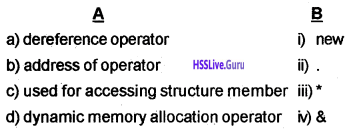a – iii
b – iv
c – ii
d – i

Question 7.
Complete the following table. The table is differentiating array and structure.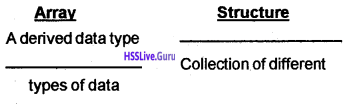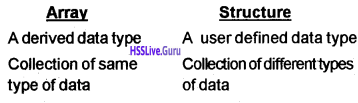Question 8.
Identify the errors in the following code fragment and correct it.
struct sample
{
int x;
float y;
} S1 ,S2;
void main()
{Struct test
int a;
float 6;
}T1,T2;
T1 = T2;
T1=S1;
}
The assignment T1 = S1 is not correct. It is a variable of type test and S1 is a variable of type sample. So it cannot be assigned.

Question 9.
When a C++ program is executed, the primary memory allocated for it is organized in a particular manner to deal with runtime memory allocation, function calls, variables, etc. Show a diagrammatic representation of it with brief explanation.
After compilation of C++ creates four distinct regions of memory used for distinct functions:

 STACK 3 HEAP 4 Global Program Variables Code 2 1

The first area (1) is used for storing the compiled code of the program. The second area (2) is used for storing global variables of the program. It remains in memory till the program ends. The third region (3) known as the stack is used for holding the return addresses of function calls, arguments passed to the function, etc. The last region heap is used for dynamic allocation.Question 10.
Analyze the following C++ statements and predict
the output.
int k = 4 ;
int *p ;
p = &k ;
cout<< k ;
cout<< *p;
cout<< *p++ ;
cout<< *(&k);
4, 4, 4, 4

Question 11.
A boy wrote a C++ program that uses several pointer variables out of which some are used for dynamic allocation. He did not use the ‘delete’ operator anywhere in the program. While running the program, the computer got stuck up after some time. Predict the reason for this kind of situation, in connection with the above program code
The situation is called memory leak. The memory allocated using ‘new’ should be properly deallocated using ‘delete’. If any function forgets to deallocate the memory using ‘delete’ which was allocated using the ‘new’ operator, it consumes some memory in every execution.

Thus a part of memory disappears with its every execution and it has some adverse effect on the system. This situation is called memory leak.

Question 12.
Is there any difference between structure and array? Explain.
Yes. An array can hold multiple elements of same datatype whereas a structure can hold multiple elements of different data types.

Question 13.
Write a C++ structure definition to store the student details given below:

2. Name
3. Marks in six subjects

Struct student
{
charname;
int mark;
}Question 14.
Observe the following layout of data,1. Name the data type in C++ used to represent the above data format.
2. Write the definition for implementing the above layout.

1. Structure

2. struct Account
{
int accno;
char name;
struct lastdate
{
int day;
int month;
int year;
} tdate;
float balanceamt;
}

Question 15.
Consider the following code segment.
struct student
{
int rollno;
char name;
struct date
{
int day;
int month;
int year;
} birthday;
char batch;
int score;
}
Write valid statements in C++ to accept the name and date of birth of a student.
gets(S1 .name);
S1.birthday.day=25;
S1.birthday.month=5;
S1.birthday. year=1991;

Question 16.
Why do we need structure variables, once a structure is defined in program?
Structure specification is merely a data type definition. Memory allocation does not take place during specification. To handle data, memory locations are needed. For memory allocation, variables are to be declared. Hence structure variable is essential for handling structured data in programs.

Question 17.
With an example explain how structures can be initialized.
Student stud = {“Athul”, 17,101, “XII Science”};
The above statement initializes structure variable stud of type student which has data members name, age, roll no, batch.Question 18.
Can we declare a structure within another structure? Explain.
Yes. We can declare a structure as a member of another structure. It is known as nested structure.

Question 19.
What does the following statement mean?
It is accessing the element of a nested structure. S is a structure variable that contains another structure variable dob. ‘month’ is a member of inner structure variable dob. This statement assigns a value 10 to the member month.

Question 20.
Thomas says that name of an array is a pointer by default. Do you agree with him? Justify.
Yes. C++ takes the array name as the address of its first element. That is, the name of an array is actually a pointer pointing to the first element of an array.

Question 21.
Distinguish between
int * ptr = new int (5);
int * ptr = new int ;
The first statement allocates memory of one integer to ptr and initialized it with value 5. The second statement allocates memory of 5 contiguous integers (ie. an array of 5 integers) and stores begining address in pointer ptr.

Question 22.
Give the syntax to declare an integer 2D array dy namically.
int * ptr = new int [m*n];
where m and n are rows and columns

Question 23.
The array of character pointers is generally preferred over two dimensional array of characters. Justify.
The array of character pointers is preferred. There are two reasons:
1. An array of pointers makes more efficient use of available memory by consuming lesser number of bytes to store the strings.

2. An array of pointers makes the manipulation of the strings much easier. One can easily exchange the positions of the strings in the array of pointers without actually touching their memory locations.

Question 24.
Predict the errors in the following code fragment in C++.
1. float f= 1.42;
float *a;
a = f;
2. int *p,*q;
p = q + 1;

1. a=&f is correct
2. No values assigned for p and q.

Question 25.
Define a structure in C++ to store book details such as book id, book name, price, and author name.
Structure is a group of different types of logically related data referenced by a single name,
struct book
{
int book id;
charbook_name;
float price;
chart author_name;
};Question 26.
Complete the following structure definition, to store students details with appropriate values. Students details are roll number, name and height.struct students
{
short rno;
char name;
float height;
};

Question 27.
Correct the following structure definition to represent an employee with employee code, employee name, and salary.
Employee struct
{
ecode int;
char name;
salary double;
}

Question 28.
Consider the array declaration. Assume that the first element of array is stored in 2020 location,
int a[] = {2,5,8,9,4},*p1 ,*p2;
p1 = a;
p2 = &a;
cout<< p1<< “\t”<< p2;
Predict the output and justify.
p1 = a means, the array name is the starting address of an array.
That means p1 = 2020
p2 = &a means p2 = 2020
Hence the output is 2020 2020

### Plus Two Computer Science Structures and Pointers Three Mark Questions and Answers

Question 1.
Explain the type of structure defined below and calculate the number of bytes required to store the structure variable s1. [Considerthe size of int as 4 bytes and char as 1 byte]
struct student
{
int mo, mark;
char name;
struct date
{
int dd, mm, yy;
}doj;
}s1;
A structure contains another structure, then it is called nested structure.Question 2.
Define a structure to represent employee details. Employee details are employee code, employee name, date of joining and place of employee.
struct employee
{
int empcode; ,
char name ;
struct date
{
int day;
int month;
int year;
}doj;
char place ;
};Question 3.
“Self referential structure can be used for implementing linked list”. Justify the statement.
A structure contains an element that is a pointer to the same structure is called self referential structure. Eg: struct date {
int dd, mm, yyyy;
date *ptr;
};
So self referential structure can be used for implementing linked list.

Question 4.
Write a program in C++ to copy one string to another without using strcpy() function.
#include
using name space std; intmain()
{
charstr [ ] = “computer”;
char * sptr;
sptr = str;
cout<< str<< endl;
cout<< sptr<< endl;
}
This code copies the string str to sptr;

Question 5.
Define a structure in C++ to store students’ details such as admission number, name, address. Address contains house number, street name and city name.
Structure is a group of different types of logically related data referenced by a single name,
struct student
{
char name;
struct
{
int house_no;
charstreet_name;
charcity_name;
};

Question 6.
A structure variable can be declared along with structure definition.” Justify this statement with an ex-ample.
Yes, it is possible that, a structure variable can be declared along with structure definition itself.
Syntax:
struct struet_name
{
Data members;
} structure variables;
Eg: struct date
{
intdd;
int mm;
intyyyy;
}d1,d2,d3;

Question 7.
Identify and correct the error in the following code fragment.
struct first {
int a; float b;
}S1;
struct second {
int a;
float b;
}S2;
S1 = S2;
The assignment S1 = S2 is not right. Because S1 and S2 are different structure variables. C++ does not permit different structure variable assignment.Question 8.
Write structure definitions for structures containing the following.

1. book no, book name, price
2. item no, item name, price, monthly sales for last 4 months.

1. struct book
{
long bookno;
char bookname;
float price;
};

2. struct item
{
int itemno;
char itemname;
float price;
int sale;
}

Question 9.
Assume that in a city, two football matches are going to be held. The coordinators of the match have decided to sell the tickets in two different styles. For the first match, the tickets will be sold one month in advance, but for the second one, tickets will be available from the stadium on the very same day of the match.

1. Correlate this situation with the memory allocation techniques in C++.
2. Compare the merits and demerits of the two in relation with the given context.

1. Static and dynamic memory allocation.

2. Every data and instruction data is being executed must be allocated some area in the main memory. The main memory can be allocated in two methods.

• Static memory allocation
• Dynamic memory allocation

When the amount of memory to be allocated is known in advance and memory is allocated during compilation itself, it is referred to as static memory allocation. When the amount of memory to be allocated is not known in advance and it is required to allocate memory as and when required during run time, it is known as dynamic memory allocation.

Question 10.
When a C++ program is compiled, the compiler allocates 20 bytes of memory and when the same program is executed 10 more bytes of memory were allocated

1. How do you account for these two types of memory allocations?
2. Name the operator used for the second type of memory allocation and also write the syntax.

1. Static and dynamic memory allocation.

2. ‘new’ operator is used for dynamic allocation of memory syntax,
datatype ‘pointer variable = new datatype;
eg: int *ptr = new int;Question 11.
Justify the following statements

1. In C++, the ‘delete’ operator performs the reverse action of ‘new’ operator.
2. If ‘arr’ is the name of an array, then arr++ is not a valid statement
3. If ‘k’ is a long variable with address 1001 and it is stored by a pointer variable ‘p’, then ‘p + 1 ’ will point to the location with address 1005.

1. In C++, the ‘delete’ operator performs the reverse action of ‘new’ operator. In C++, both ‘new’ and ‘delete’ operators are used for dynamic allocation and deallocation of memory respectively, ie. For allocation of memory at runtime new operator is used. For deallocation of memory that have been allocated by the new operator, ‘delete’ operator is used.

2. arr++ is not a valid statement. Because the base address of an array can not change. Array name gives the base address of the array.

3. Pointer variable hold the address of memory location where the data is stored. So incrementing the pointer variable is adding the size of the data type. Long data type takes 4 bytes of memory. So incrementing the pointer variable P makes it point to the address 1005.

Question 12.
Discuss the similarity and differences of a structure as compared to an array and a class.

 Structures Array 1. It is a user defined data type 1. Predefined data type 2. It is a collection of different types of logically related data under one name. 2. Collection of data elements of same data type having a common name 3. Elements referenced using dot operator (.) 3. Elements references using its subscripts (position value) 4. When an element of a structure becomes another structure nested structure and complex structures are formed 4. When an element of another becomes another array, multidimensional arrays are formed. 5. Structures contain array as its elements 5. Array of structure can be formed

Question 13.
Considerthe following code fragment.
char nam[ ] = “COMPUTER”
for(int i = 0; nam[i]!= ‘\0’; i++)
cout << nam[i];
Rewrite the above code to get the same output using a character pointer variabe.
char nam [ ] = “computer”
char*P;
for (P = nam; *P!= ‘\0’; P++)
cout<< *P;Question 14.
Match the following.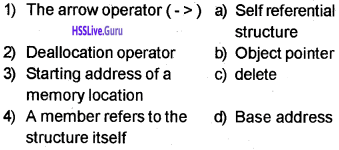1 – b
2 – c
3 – d
4 – a

Question 15.
How is dynamic memory allocation different from static memory allocation?
In the static memory allocation, the amount of memory to be allocated is predicted and pre known. This memory is allocated during the compilation itself. All the declared variables declared normally, are allocated memory statically.

In the dynamic memory allocation, the amount of memory to be allocated is not known beforehand. It is allocated during run time as and when required. The memory is dynamically allocated using new1 operator.

The objects that are allocated memory statically have the lifetime as their scope allows, as decided by the compiler. And the objects that are allocated memory dynamically have the lifetime as decided by the programmer. That is until the programmer explicitly deallocates the memory, such objects live in the memory.

### Plus Two Computer Science Structures and Pointers Five Mark Questions and Answers

Question 1.
struct Book
Computer Science
{
int book_no;
charbk_name ;
struct
{
short dd;
short mm;
short yy;
} dt_of_purchase;
float price;
};
a) Write a C++ statement to declare a variable to refer to the details of a book. What is the memory requirement of this variable? Justify your answer.

b) Write a C++ statement to initialize this variable with the details of your Computer Science textbook.

c) Write C++ statement (s) to display the details of the book.

d) The missing of structure tag in the inner structure does not cause any error. state whether this is true or false. Give reason.
a) Book b;
The memory requirements are as follows: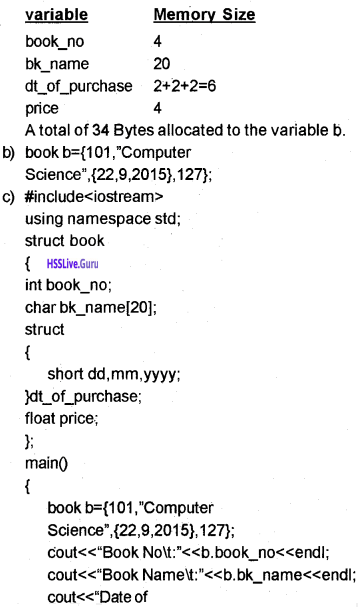d) This is true. There is no need to mention the structure tag because it is declared inside the structure.Question 2.
You are given an assignment by the teacher to write a C++ program that accepts the age of ’n’ people who attended a medical camp. The value of ‘n’ is not known beforehand. Its value is to be accepted at the time of running the program. The program should print the average age as the final output. Develop the code.Question 3.
How are the following related?

1. Structure and array
2. Structure and class